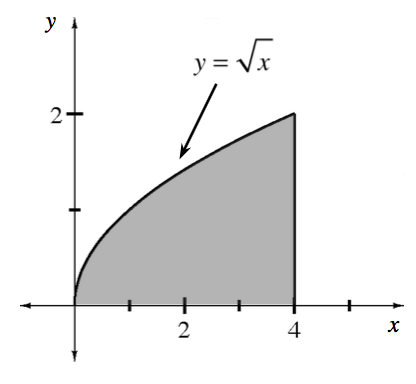### Home > APCALC > Chapter 8 > Lesson 8.1.5 > Problem8-51

8-51.

Calculate the volume of the region bounded by $y =\sqrt { x }$, $y = 0$, and $x = 4$ revolved about each of the lines below. Use the steps outlined in problem 8-49.

1. $x$-axis

After sketching an arbitrary rectangle (with $Δx→0$), it is apparent that there will not NOT be a hole in the center of this solid.
That indicates that the Disk Method will be appropriate.

2. $y$-axis

This arbitrary rectangle indicates that there will be a hole in the center of this solid. You will have to use the Washer Method.

Horizontal rectangles indicate that the bounds and the integrand will be in terms of $y$.

Notice that the figure will be a cylinder with a curvy hole in the center.
The radii of the cylinder is $R = 4$.
The radii of the curvy hole is $r = y^2$.

3. $x = −2$

Q: Washers or disks? Think: Will there be a hole or won't there?

Q: Will the integral be in terms of $x$ or $y$? Think: Are the rectangles vertical or horizontal?

Notice that these radii are $2$ units longer than the radii in part (b).
$R = 2+4$
$r = 2 + y^2$

4. $y = 5$

$R = 5$

Since $y=\sqrt{x}$ represents the distance between the $x\text{-axis and }y=\sqrt{x}$, it follows that:$r=5-\sqrt{x}$